Selected writings
contact

# Approximate derivation of critical buckling load

Jonathan Ochshorn

Euler's famous equation for critical buckling load is rather cumbersome to derive, relying as it does on solving a second order differential equation of the form:

EI(d2y / dx2) + Py = 0.

The simplified and approximate derivation proposed here is not intended to replace Euler's brilliant work, but rather is offered as a supplementary analysis that may lend some insight into the behavior of axially-loaded columns. To anticipate the results: one can get within 3% of Euler's critical buckling load for a pin-ended column of length, L, simply by dividing the mid-span moment of a uniformly-loaded simply-supported beam of the same length by its mid-span deflection. Here's why.

Buckling occurs when a second state of equilibrium becomes possible for a given load on a column; i.e., when in addition to the obvious condition (Fig. 1-a) of axial loading, we get a second possible state of equilibrium (Fig. 1-b) with a deflected, or buckled, shape.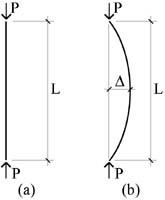Fig. 1 Alternate states of equilibrium: (a) for straight axially-loaded column; and (b) for buckled column.

The basis for this second possible state of equilibrium is that the resisting moments in the deflected column are consistent with the moments caused by the axial load in relation to the deflected shape. One way of looking at this is to imagine that the column is first pulled into a deflected shape by some supernatural power (or just by our powers of imagination: see Fig. 2).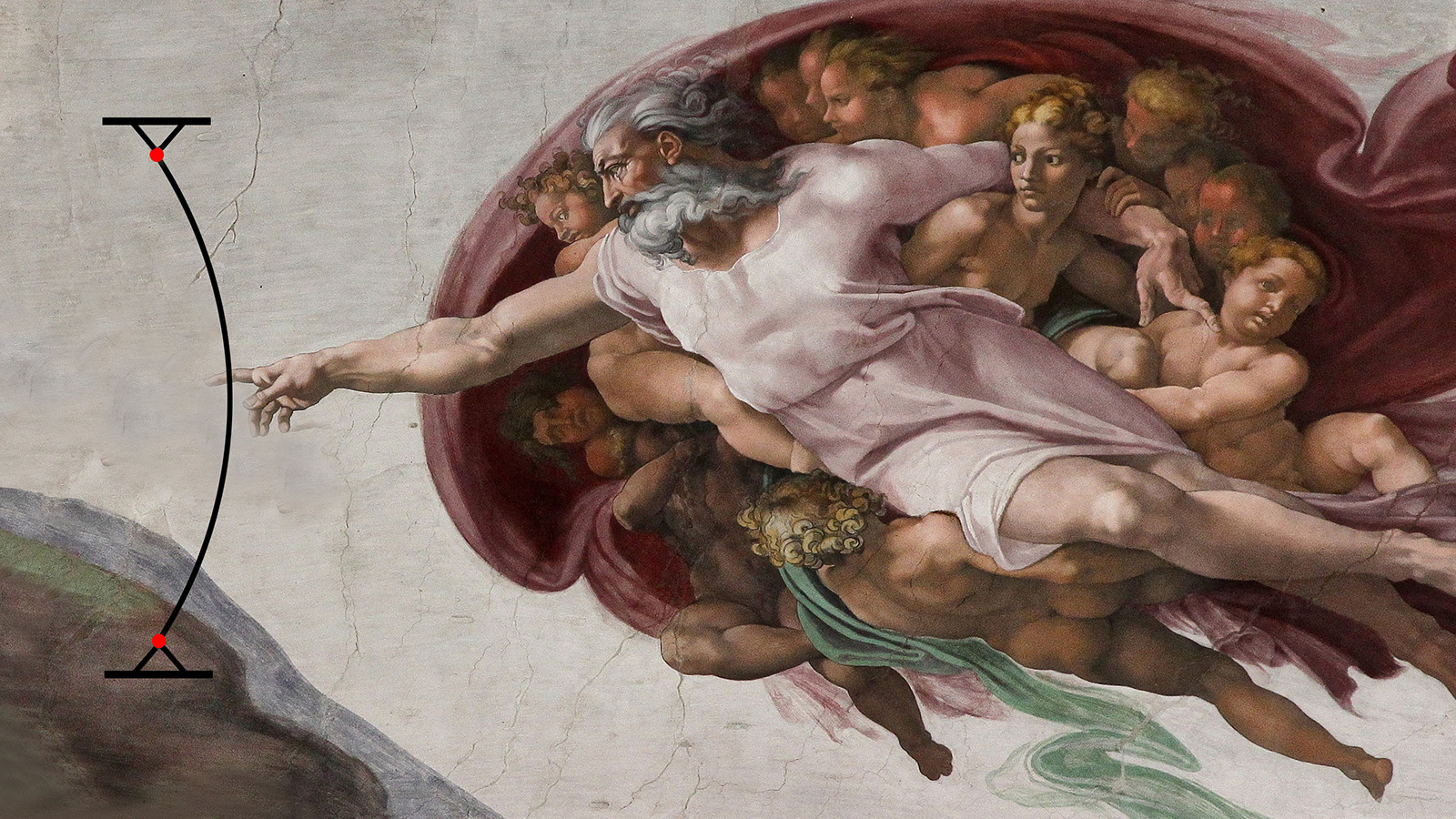Fig. 2 Column pulled into a deflected position (with apologies to Michelangelo).

The column, having been stretched and compressed elastically, would attempt to regain (spring back to) its initial undeflected shape were it not for our ability to temporarily hold it in its deflected position. Now, if we quickly replace our own "supernatural" force with an axial force on the column, but leave the deflected shape as it was, we generate a bending moment due to the misalignment of the applied axial force with the internal axial forces (Fig. 3). If this moment due to the axial force is smaller than the moment caused by our initial (supernatural or imaginary) misalignment — smaller because the axial load, P, is not big enough to match the resisting moment — equilibrium cannot be maintained and the column will spring back elastically to its original (straight) position. However, if the moments caused by the applied axial load are consistent with those moments originally applied by the prior (supernatural) loading, then equilibrium is maintained, and the column can be said to have buckled.Fig. 3 Moment caused by deflection of axially-loaded column.

The axial load, P, that establishes this equilibrium is the critical buckling load, since any small misalignment will move the column inexorably from the ideal axial condition (Fig. 1-A) to the buckled state (Fig. 1-B). Based on the approximate method outlined here (as well as Euler's), this load is consistent with any deflection, so that the safety of such a column would be immediately threatened when that load is approached.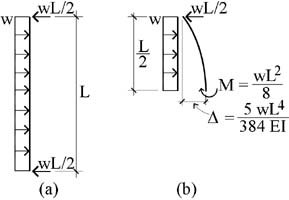Fig. 4 Column uniformly loaded: (a) like a simply-supported beam; and (b) free-body diagram of column cut at mid-span.

To calculate the critical buckling load, Pcr, the axial load, P, is replaced with a uniformly-distributed load, w, acting along the column's length as if it were a vertical beam (Fig. 4-a). Under this loading, the mid-span moment and deflection are as follows (Fig. 4-b):*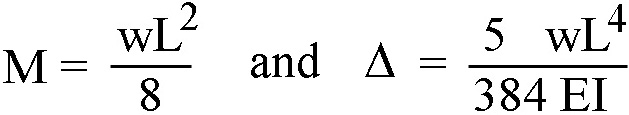Fig. 5 Free-body diagram for buckled column.

That being said, we can still find the approximate buckling load corresponding to these "beam" values, even if no compatible deflected shape can be found. As before, rotational equilibrium for the critical buckling load (Fig. 3) is defined by Pcr = M / Δ.

Substituting the familiar "beam" values for M and Δ (Fig. 4-b) into this equation, we get: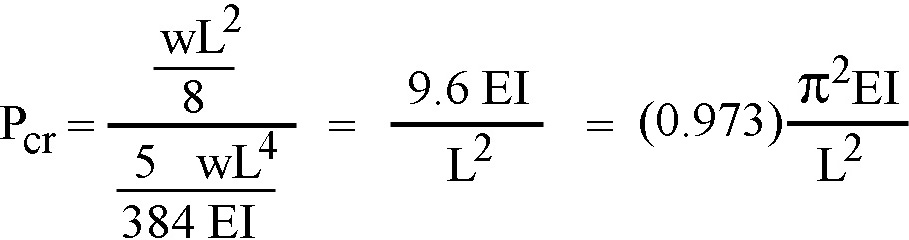This is quite close (and slightly conservative) compared to Euler's equation:Because all applied loads have cancelled out of these equations, the deflection associated with Pcr is indeterminate: in other words, since any deflection is consistent with this critical buckling load, the safety of such a column is fatally compromised once this load is reached.

It should be noted that even Euler's famous equation is not exact. His exact equation for buckling, rarely used in practice, shows that a point of catastrophic and sudden buckling never actually occurs.*** Rather, equilibrium may be maintained with increasing loads corresponding to increasing deflections (beyond the critical buckling load of the approximate equation), up until the point where the material's ultimate strength is exceeded and failure occurs under combined axial and bending stresses. However, deflections do increase quite rapidly once the approximate critical buckling load is reached, so that these approximate equations serve as an entirely rational indicators of the "point of no return" for the initiation of buckling.